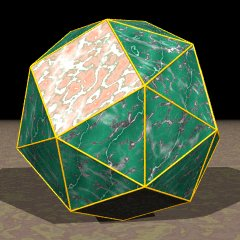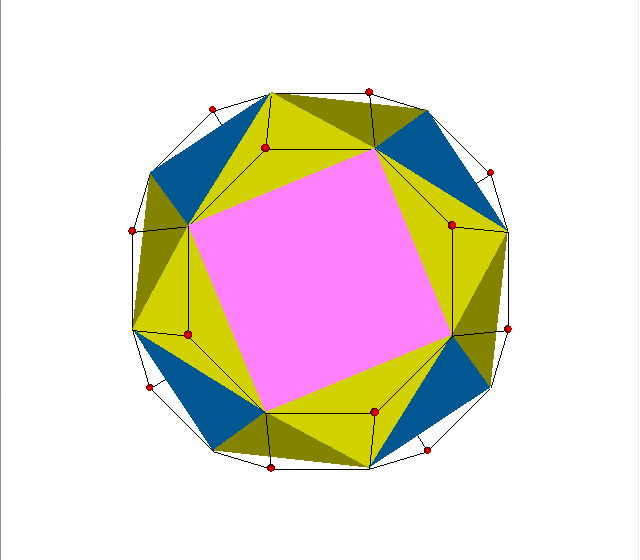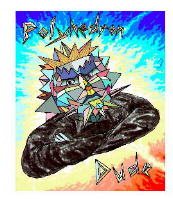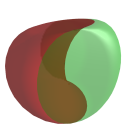Acronym snic TOCID symbol sCO Name snub cube,snub cuboctahedron `© ©` Circumradius sqrt[(10+cbrt[199+3 sqrt(33)]+cbrt[199-3 sqrt(33)])/12] = 1.343713 Vertex figure [34,4] Snub derivation `` Coordinates (C1, C2, C3)   & all even permutations, all even changes of sign   & all odd permutations, all odd changes of sign where C1 = sqrt[(4-c1+c2)/12] = 0.337754, C2 = sqrt[(2+c1-c2)/12] = 0.621226, C3 = sqrt[(4+c3+c4)/12] = 1.142614, and c1 = cbrt[3 sqrt(33)+17], c2 = cbrt[3 sqrt(33)-17], c3 = cbrt[199+3 sqrt(33)], c4 = cbrt[199-3 sqrt(33)] General of army (is itself convex) Colonel of regiment (is itself locally convex – no other uniform polyhedral members) Dihedral angles between {3} and {3}:   arccos([1-2 cbrt(19+3 sqrt(33))-2 cbrt(19-3 sqrt(33))]/9) = 153.234588° between {3} and {4}:   arccos(-sqrt([cbrt(19+3 sqrt(33))+cbrt(19-3 sqrt(33))-1]/[cbrt(19+3 sqrt(33))+cbrt(19-3 sqrt(33))+1])) = 142.983430° Confer compounds: disco   diminishings: odsnic ExternallinksIncidence matrix according to Dynkin symbol

```s3s4s

demi( . . . ) | 24 |  1  2  2 | 1 1  3
--------------+----+----------+-------
s 2 s   ♦  2 | 12  *  * | 0 0  2
sefa( s3s . ) |  2 |  * 24  * | 1 0  1
sefa( . s4s ) |  2 |  *  * 24 | 0 1  1
--------------+----+----------+-------
s3s .   ♦  3 |  0  3  0 | 8 *  *
. s4s   ♦  4 |  0  0  4 | * 6  *
sefa( s3s4s ) |  3 |  1  1  1 | * * 24

starting figure: x3x4x
```